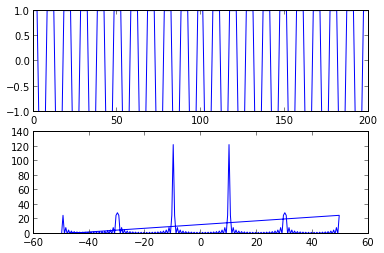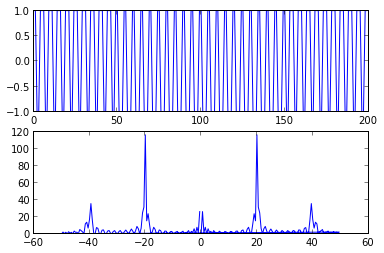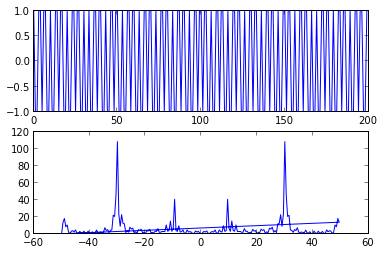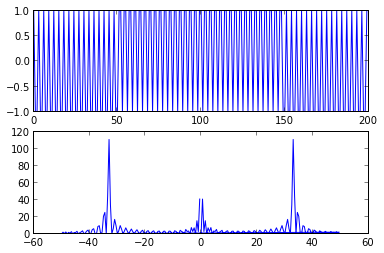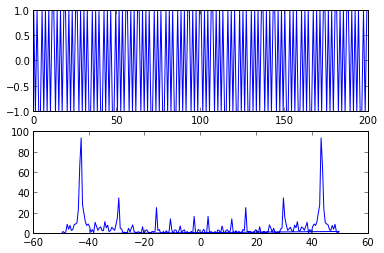In :
def plotspec(x, Ts):
fig = figure()
ax1.plot(x)

q = fft.fft(x)
ax2.plot(fft.fftfreq(len(x), Ts), abs(q))

def arbspec(s, time, Ts):
t = linspace(0.0, time, time/Ts)
x = s(t)
plotspec(x, Ts)

def speccos(f, phi, time, Ts):
def s(t):
return cos(2*pi*f*t+phi)
arbspec(s, time, Ts)


3.11. With a sample rate of 100Hz, plot the spectrum of cosine waves with f=30,40,49,50,51,60Hz. Which show aliasing?

In :
for f in [30,40,49,50,51,60]:
speccos(f, 0, 2.0, 1.0/100.0)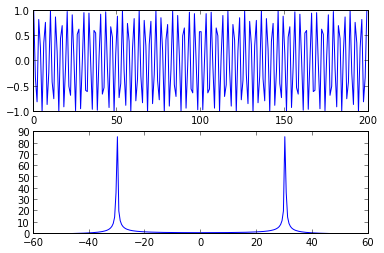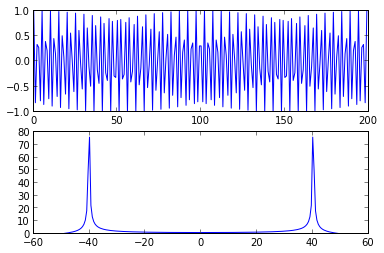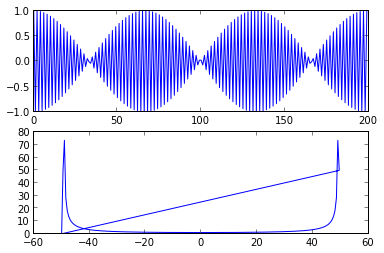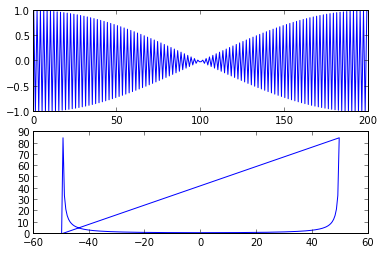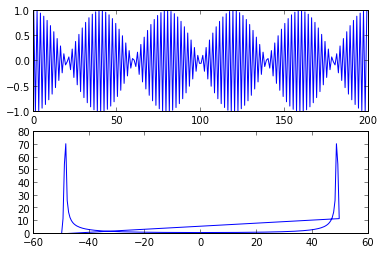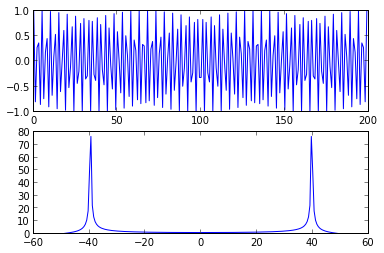3.12. Creating a cosine wave with f=50Hz, plot the spectrum with sampling rates of 50,90,100,110,200 Hz. Which show aliasing?

In :
for Fs in [50,90,100,110,200]:
speccos(50.0, 0, 2.0, 1.0/float(Fs))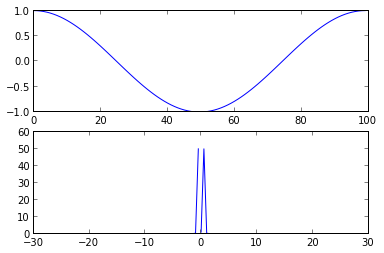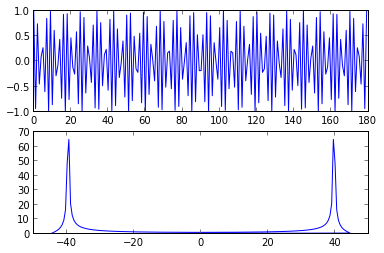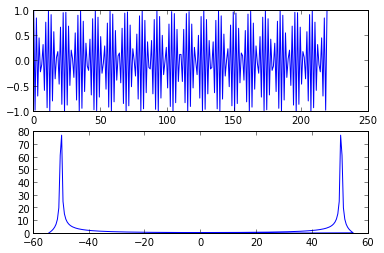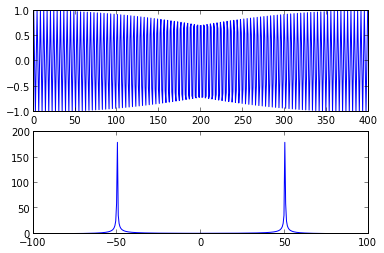3.13. Mimic the code in speccos.m with sampling interval Ts=1/100 to find the spectrum of square waves with fundamental f=10,20,30,33,43 Hz. Can you predict where the spikes will occu

In :
def specsquare(f, time, Ts):
t = linspace(0.0, time, time/Ts) # Create a time vector
x = sign(cos(2*pi*f*t)) # Square wave = sign of cos wave
plotspec(x, Ts)

Ts = 1.0/100.0

for f in [10,20,30,33,43]:
specsquare(f, 2.0, Ts)